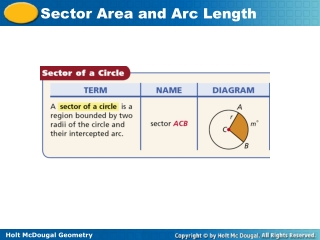DownloadDownload PresentationTélécharger la présentation- - - - - - - - - - - - - - - - - - - - - - - - - - - E N D - - - - - - - - - - - - - - - - - - - - - - - - - - -
##### Presentation Transcript

1. Helpful Hint Write the degree symbol after m in the formula to help you remember to use degree measure not arc length.

2. Example 1A: Finding the Area of a Sector Find the area of each sector. Give answers in terms of and rounded to the nearest hundredth. sector HGJ Use formula for area of sector. Substitute 12 for r and 131 for m. Simplify. = 52.4 m2  164.62 m2

3. Example 1B: Finding the Area of a Sector Find the area of each sector. Give answers in terms of and rounded to the nearest hundredth. sector ABC Use formula for area of sector. Substitute 5 for r and 25 for m. Simplify.  1.74 ft2  5.45 ft2

4. Check It Out! Example 1a Find the area of each sector. Give your answer in terms of  and rounded to the nearest hundredth. sector ACB Use formula for area of sector. Substitute 1 for r and 90 for m. Simplify. = 0.25 m2  0.79 m2

5. Check It Out! Example 1b Find the area of each sector. Give your answer in terms of  and rounded to the nearest hundredth. sector JKL Use formula for area of sector. Substitute 16 for r and 36 for m. Simplify. = 25.6 in2  80.42 in2

6. Example 2: Automobile Application A windshield wiper blade is 18 inches long. To the nearest square inch, what is the area covered by the blade as it rotates through an angle of 122°? Use formula for area of sector. r = 18 in.  345 in2 Simplify.

7. Check It Out! Example 2 To the nearest square foot, what is the area watered in Example 2 (p. 765) as the sprinkler rotates through a semicircle? Use formula for area of sector. d = 720 ft, r = 360 ft  203,575 ft2 Simplify.